# Samacheer Kalvi 9th Maths Solutions Chapter 4 Geometry Ex 4.7

## Tamilnadu Samacheer Kalvi 9th Maths Solutions Chapter 4 Geometry Ex 4.7

Multiple Choice Questions :

Question 1.
The exterior angle of a triangle is equal to the sum of two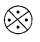(1) Exterior angles
(2) Interior opposite angles
(3) Alternate angles
(4) Interior angles
Hint: Exterior angle = 180°- Interior angle = sum of interior opposite angle
Solution:
(2) Interior opposite angles

Question 2.
In the quadrilateral ABCD, AB = BC and AD = DC Measure of ∠BCD is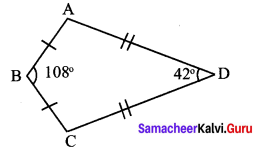(1) 150°
(2) 30°
(3) 105°
(4) 72°
Hint: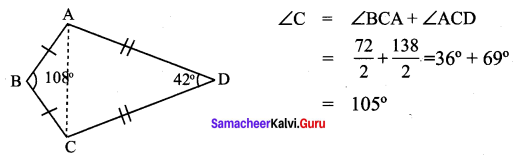Solution:
(3) 105°

Question 3.
ABCD is a square, diagonals AC and BD meet at O. The number of pairs of congruent triangles are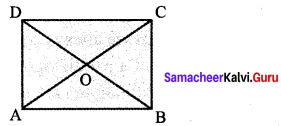(1) 6
(2) 8
(3) 4
(4) 12
Solution:
(1) 6

Question 4.
In the given figure CE || DB then the value of x° is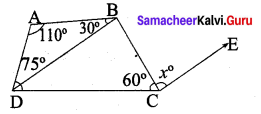(1) 45°
(2) 30°
(3) 75°
(4) 85°
Hint: 35° + x°+ 60° = 180° ⇒ x = 85°
Solution:
(4) 85°

Question 5.
The correct statement out of the following is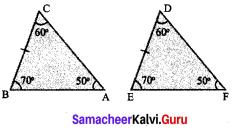(1) ∆ABC ≅ ∆DEF
(2) ∆ABC ≅ ∆DFE
(3) ∆ABC ≅ ∆FDE
(4) ∆ABC ≅ ∆FED
Hint: ∠C = ∠D; ∠B = E; ∠A = ∠F
Solution:
(4) ∆ABC = ∆FED

Question 6.
If the diagonal of a rhombus are equal, then the rhombus is a
(1) Parallelogram but not a rectangle
(2) Rectangle but not a square
(3) Square
(4) Parallelogram but not a square
Solution:
(3) Square

Question 7.
If bisectors of ∠A and ∠B of a quadrilateral ABCD meet at O, then ∠AOB is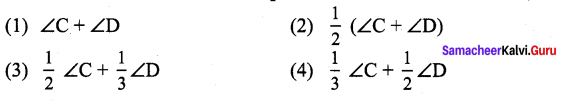Hint: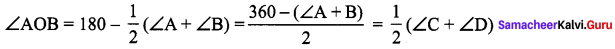Solution:
(2) $$\frac{1}{2}(\angle \mathbf{C}+\angle \mathbf{D})$$

Question 8.
The interior angle made by the side in a parallelogram is 90° then the parallelogram
is a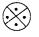(1) rhombus
(2) rectangle
(3) trapezium
(4) Kite
Hint:
If one angle of a parallelogram is 90°, then it is a rectangle
Solution:
(2) rectangle

Question 9.
Which of the following statement is correct?
(1) Opposite angles of a parallelogram are not equal.
(2) Adjacent angles of a parallelogram are complementary.
(3) Diagonals of a parallelogram are always equal.
(4) Both pairs of opposite side of a parallelogram are always equal.
Hint:
Opposite sides of a parallelogram are equal.
Solution:
(4) Both pairs of opposite side of a parallelogram are always equal

Question 10.
The angles of the triangle are 3x – 40, x + 20 and 2x – 10 then the value of x is
(1) 40°
(2) 35°
(3) 50°
(4) 45°
Hint: 3x – 40 + x + 20 + 2x- 10 – 180° ⇒ 6x = 210 ⇒ x = 35°
Solution:
(2) 35

Question 11.
PQ and RS are two equal chords of a circle with centre O such that ∠POQ = 70°, then ORS =
(1) 60°
(2) 70°
(3) 55°
(4) 80°
Solution:
(3) 55°
Hint: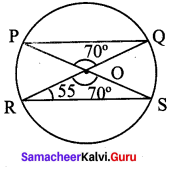Question 12.
A chord is at a distance of 15cm from the centre of the circle of radius 25cm. The length of the chord is
(1) 25 cm
(2) 20 cm
(3) 40 cm
(4) 18 cm
Hint: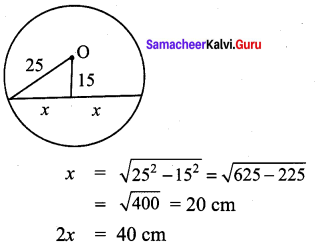Solution:
(3) 40 cm

Question 13.
In the figure, O is the centre of the circle and ∠ACB = 40° then ∠AOB =
(1) 80°
(2) 85°
(3) 70°
(4) 65°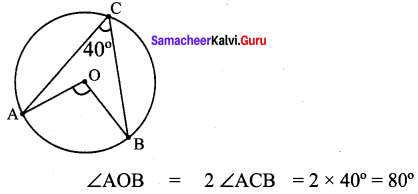Hint: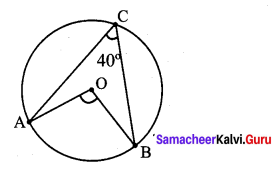Solution:
(1) 80°

Question 14.
In a cyclic quadrilaterals ABCD, ∠A = 4x, ∠C = 2x the value of x is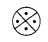(1) 30°
(2) 20°
(3) 15°
(4) 25°
Hint: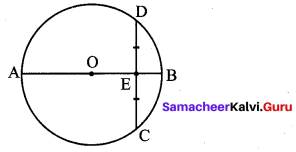Solution:
(1) 30°

Question 15.
In the figure, O is the centre of a circle and diameter AB bisects the chord CD at a point E such that CE = ED = 8 cm and EB = 4 cm. The radius of the circle is
(1) 8 cm
(2) 4 cm
(3) 6 cm
(4) 10 cm
Hint: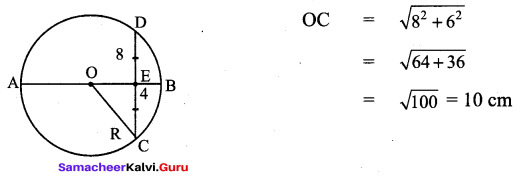Solution:
(4) 10 cm

Question 16.
In the figure, PQRS and PTVS are two cyclic quadrilaterals, if ∠QRS = 80°, then ∠TVS =
(1) 80°
(2) 100°
(3) 70°
(4) 90°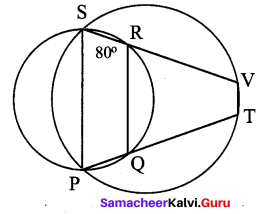Hint: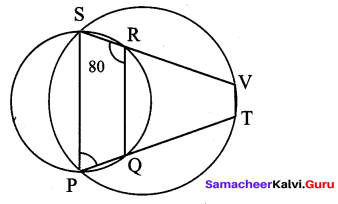Solution:
(1) 80°

Question 17.
If one angle of a cyclic quadrilateral is 75°, then the opposite angle is
(1) 100°
(2) 105°
(3) 85°
(4) 90°
Hint: 180° – 75° =105°
Solution:
(2) 105°

Question 18.
In the figure, ABCD is a cyclic quadrilateral in which DC produced to E and CF is drawn parallel to AB such that ∠ADC = 80° and ∠ECF = 20°, then ∠BAD = ?
(1) 100°
(2) 20°
(3) 120°
(4) 110°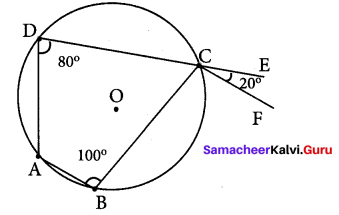Hint: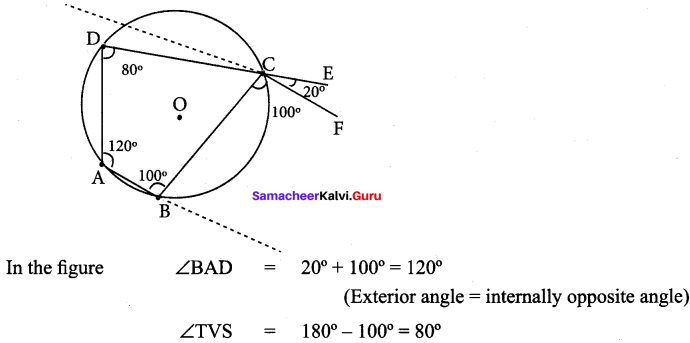Solution:
(3) 120°

Question 19.
AD is a diameter of a circle and AB is a chord. If AD = 30 cm and AB = 24 cm then the distance of AB from the centre of the circle is
(1) 10 cm
(2) 9 cm
(3) 8 cm
(4) 6 cm
Hint: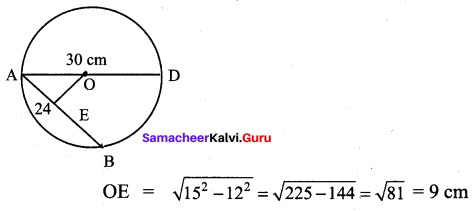Question 20.
In the given figure, If OP = 17cm PQ = 30 cm and OS is perpendicular to PQ, then RS is
(1) 10 cm
(2) 6 cm
(3) 7 cm
(4) 9 cm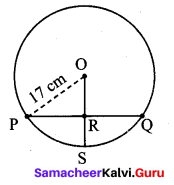Hint: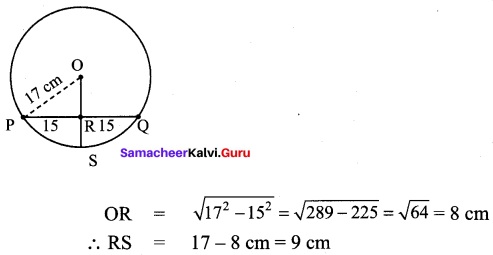Solution:
(4) 9 cm